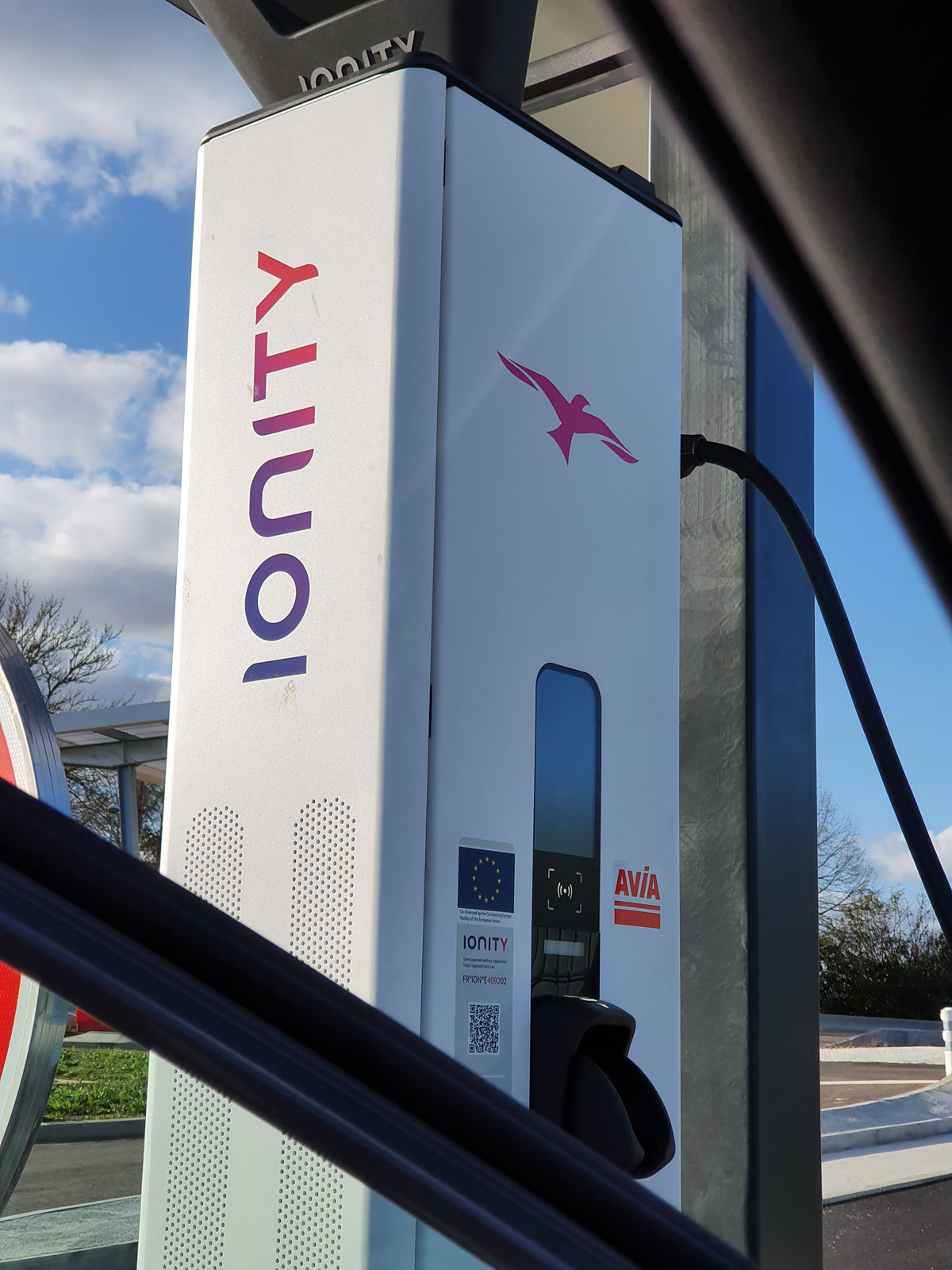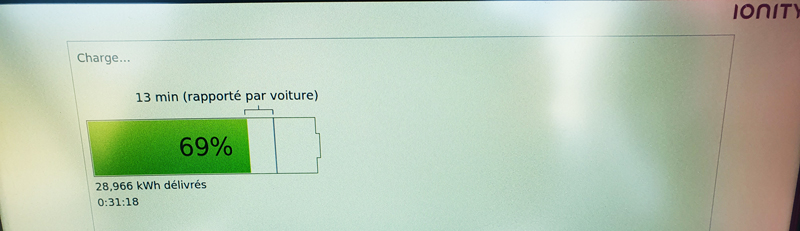# The abbreviations kW, kWh, MWh and kVA

In the field of electric vehicles, it is useful to master a few terms related to electricity: kW, kWh or even MWh and kVA.
Rather than going off on a run and saying “…it’s too complicated for me…”, here are some simple explanations to make it easier for newcomers to electric mobility.## The kilowatt or kW and the kilovolt-ampere or kVA

An electric vehicle, like any other electrical device, needs energy to run.
The power needed to drive different devices is different for one and another. The unit to measure this power is the watt and its multiples the kilowatt (kW), the megawatt (MW), etc.
So, we speak of power in kW (kilowatts). One kilowatt (kW) is equal to 1000 watts and the megawatt (MW) is equal to one million watts (1000 kW).
The kilovoltampere or kVA is also sometimes used to express the power of a device, which is equivalent to one kilowatt. Therefore, simply remember that 1 kVA = 1 kW.

## The kilowatt-hour or kWh

The kilowatt-hour, kilowatt-hour or kWh represents a quantity of energy consumed during one hour.
1 kWh is the amount that a 1 kW electrical device will consume if it is operated for one hour.
The capacity of batteries in electric vehicles is therefore also expressed in kilowatt-hours (kWh).
The battery of an electric car with a capacity of 50 kWh will therefore theoretically be able to provide 1 kW of power for 50 hours, before it is completely empty.## Confusion between kilowatts and kilowatt-hours

These two units are often confused, while they refer to something completely different.
Quite abstract for newcomers to electric mobility, here’s a simple example to better understand:

Confusing the kilowatt (kW) with the kilowatt-hour (kWh) is like confusing for example
kilometres (km) with kilometres per hour (km/h): so a distance with a speed.

The power of a charging station for electric vehicles is therefore expressed in kW (kilowatts), while the amount of energy stored during battery recharging is expressed in kWh (kilowatt-hours).

Scroll to Top